# Mathematics 1010 online

## Intervals

Intervals are chunks of the real line. Specifically we define for real numbers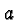and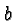:

•: the set of all numbers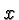satisfying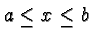.
•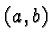: the set of all numberssatisfying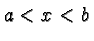.

•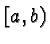: the set of all numberssatisfying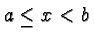.

•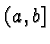: the set of all numberssatisfying.

So, for example, the intervalis the set of all numbers greater than or equal to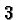and less than. The numbers,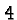, and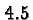are in the interval, the numbers,and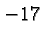are not.

The notation may be a little confusing, but just remember that square brackets mean the end point is included, and round parentheses mean it's excluded. If both end points are included the interval is said to be closed, if they are both excluded it's said to be open. If one is included and the other excluded the interval is half open (or half closed, depending on your preference).

Now things get a little murky because the above notation is also used withreplaced with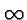orreplaced with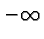(and only round parentheses at that end). This means that the interval is unlimited on the right or left, respectively.

For example the notation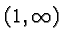is a fancy way of describing the set of all numbers greater than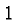, and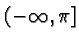means the set of all number less than or equal to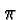. The set of all real numbers can be expressed as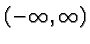.